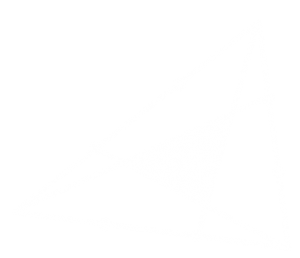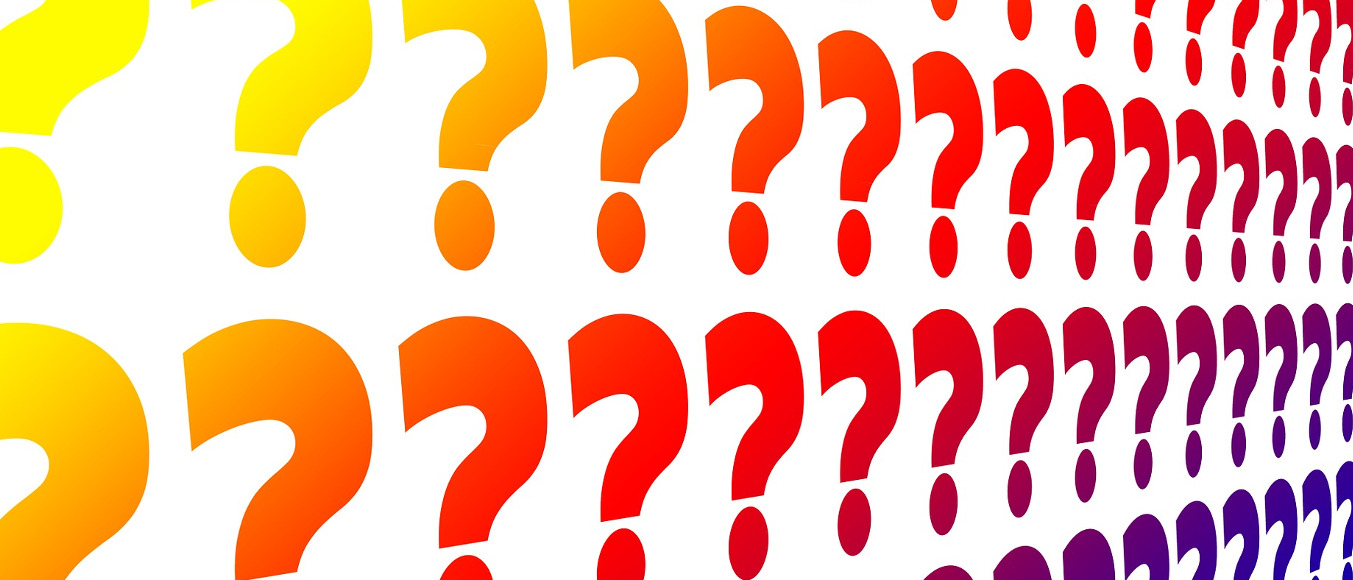# Puzzles, Issue 01

These puzzles appeared in Issue 01 of the magazine.

### #2 – CirclesSource: MathsJam
Pictured are: a quarter circle with radius 2r, and two semi circles with radius r.
Prove that the pink area is equal to the green area.

### #104 – Two TrianglesSource: MathsJam
In the diagram, the three sides of a triangle have been split into thirds, then the lines have been added.
What is the area of the smaller shaded triangle as a fraction of the area of the large triangle?

The answers to these puzzles are available here.These puzzles appeared in Issue 01 of the magazine.

### #2 – CirclesSource: MathsJam
Pictured are: a quarter circle with radius 2r, and two semi circles with radius r.
Prove that the pink area is equal to the green area.

### #104 – Two TrianglesSource: MathsJam
In the diagram, the three sides of a triangle have been split into thirds, then the lines have been added.
What is the area of the smaller shaded triangle as a fraction of the area of the large triangle?

The answers to these puzzles are available here.